# WolframCalculus & AlgebraA Core Part of the Wolfram Language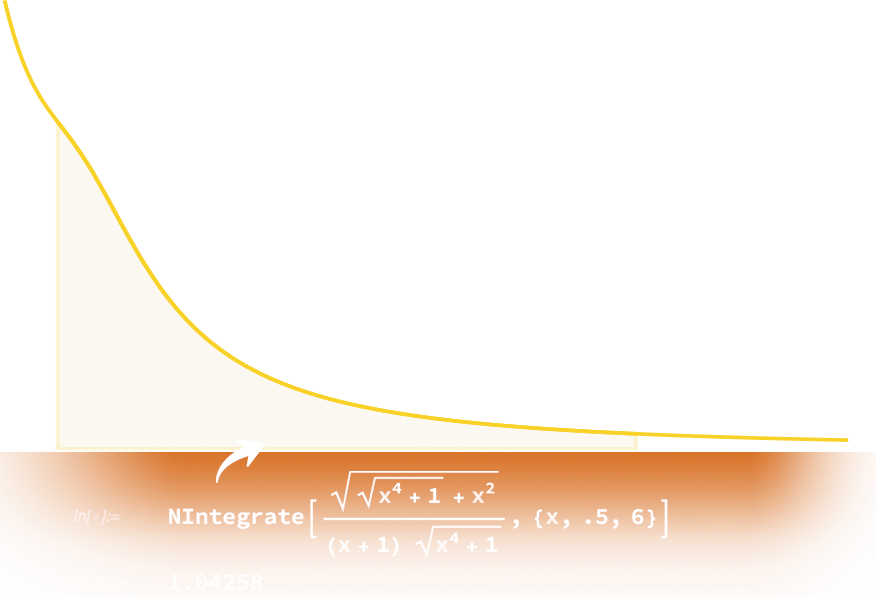Symbolic & Numeric Mathematical Computation. For everyone from middle-school students to advanced math researchers. Centuries of mathematical development packed into a set of exceptionally powerful functions tightly integrated with advanced visualization and immediately computable built-in data.## Elementary & Polynomial Algebra

Factor and expand polynomials; manipulate, combine and simplify equations and inequalities; and solve systems of equations exactly or to any desired numerical precision. Use step-by-step operations to help master foundational material or use advanced algorithms to solve complicated equations.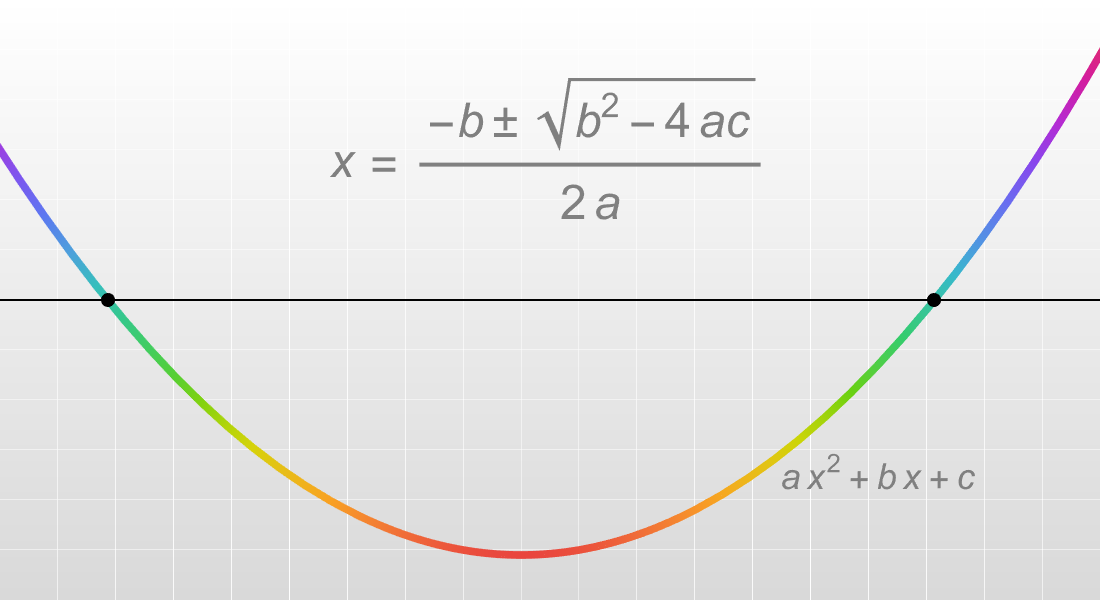## Calculus

Compute limits, derivatives, integrals and other concepts in calculus using a combination of powerful symbolic and numeric methods for optimal results.

## Differential Equations

Solve ordinary, partial, delay, integral and hybrid differential equations. Differential equations are a key modeling tool for science and engineering. Use the solutions to predict behavior and understand dependence on parameters.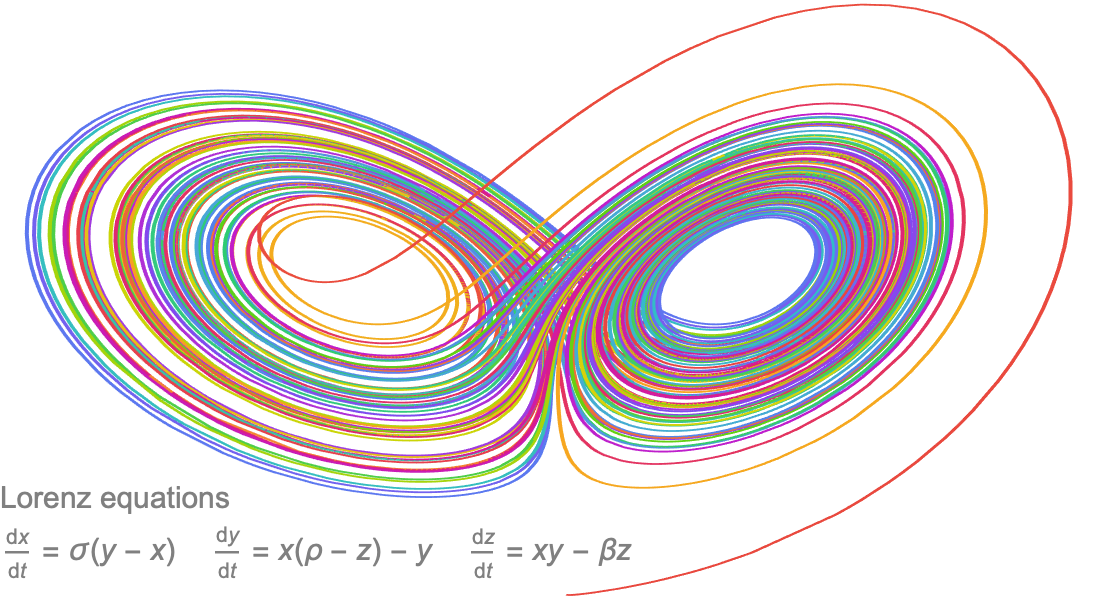## Multivariate & Vector Calculus

Explore core concepts in geometry, PDEs, physics and related fields. Easily compute multidimensional limits (scalar and vector), derivatives (partial, gradient, divergence, etc.) and integrals (line, surface, volume, etc.). Visualize computed vector fields and solutions.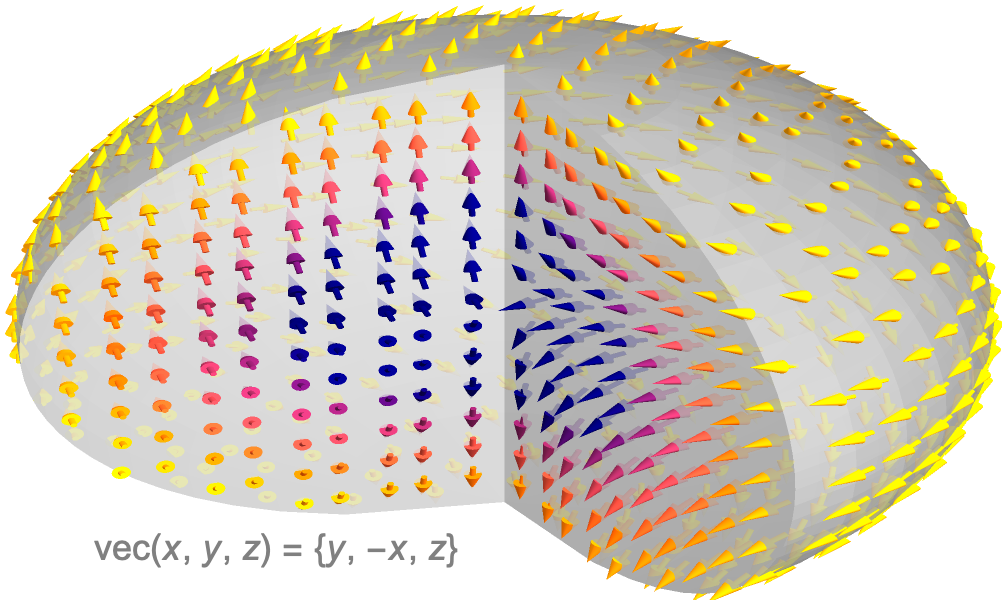## Linear Algebra

Automatically solve linear systems, compute matrix eigendecompositions and analyze data with SVD. Comes with a full stack of linear algebra functionality, including numeric and symbolic dense, sparse and structured methods. Used extensively in data science, statistics, optimization and geometry.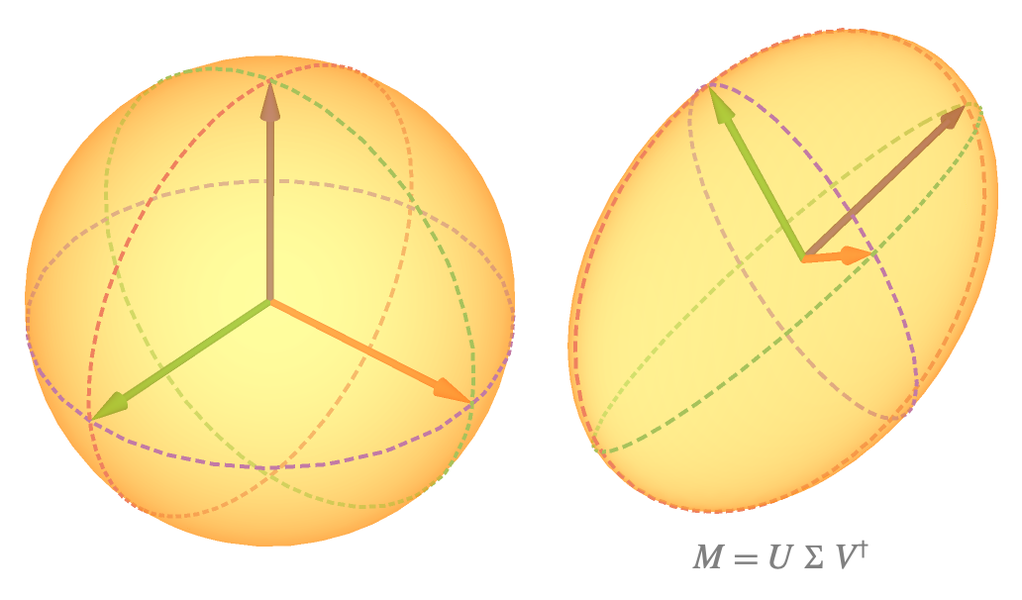## Mathematical Functions

Access a comprehensive collection of dedicated functions from physics, engineering, statistics and other fields. Elementary, Bessel, elliptic, hypergeometric and other functions are well integrated and can be visualized, symbolically simplified or evaluated to any numeric precision.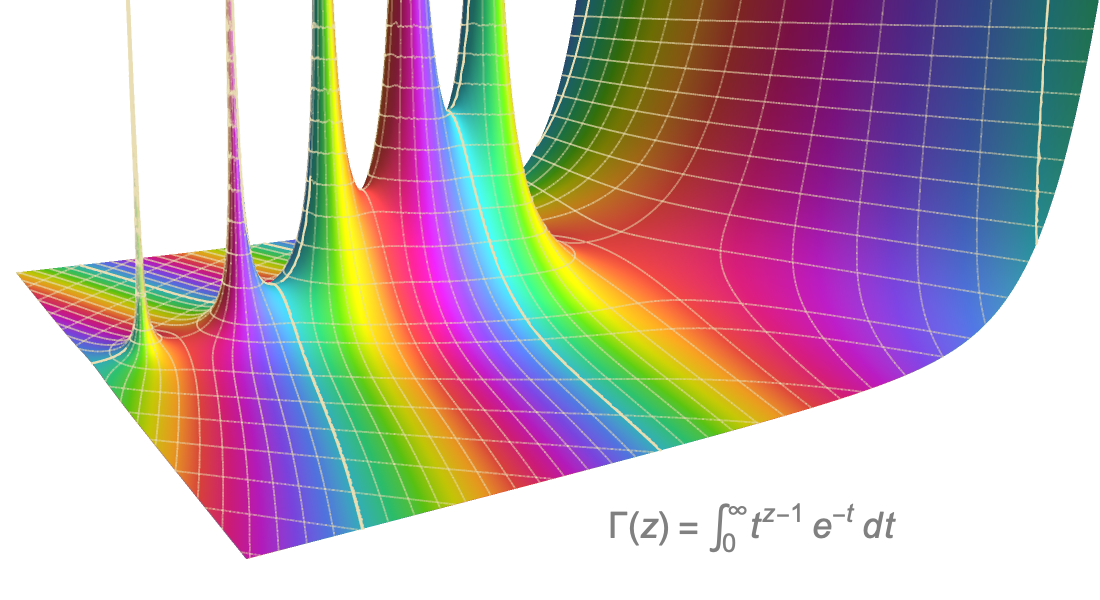## Discrete Calculus

Solve fundamental problems in analysis of algorithms, numerical analysis and combinatorics, such as computing finite and infinite sums and products or solving difference and recurrence equations. Leverage a full set of functions backed by modern methods.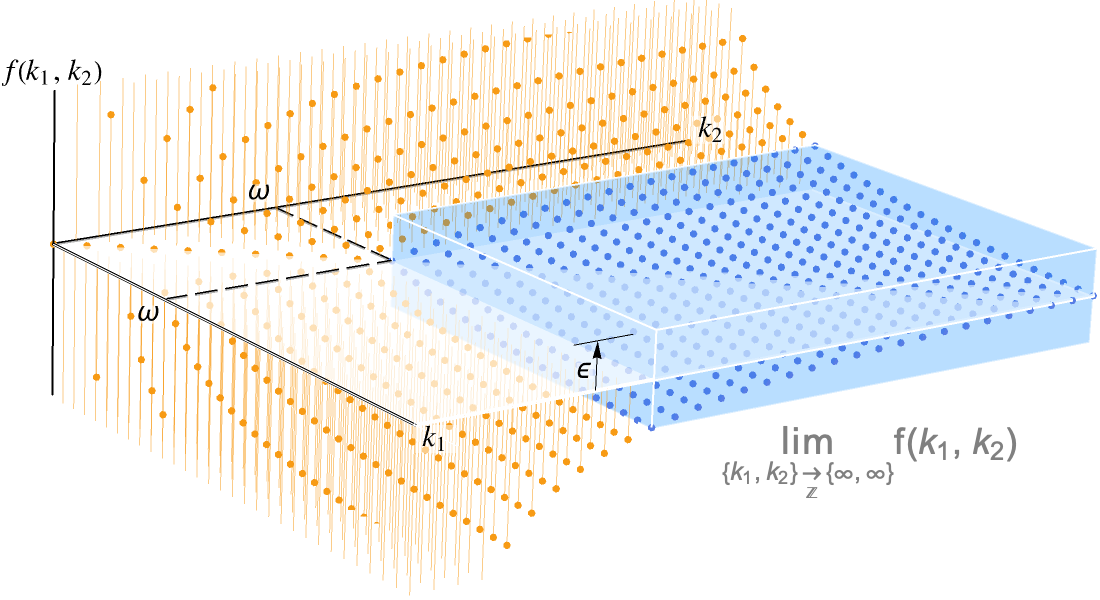## Asymptotic Calculus

Use asymptotic methods as a complement to exact symbolic and numeric computations to find approximate solutions to hard problems. A comprehensive set of automated solvers can be used in a wide variety of areas, including number theory, analysis of algorithms, probability and statistics, theoretical physics and numerical analysis.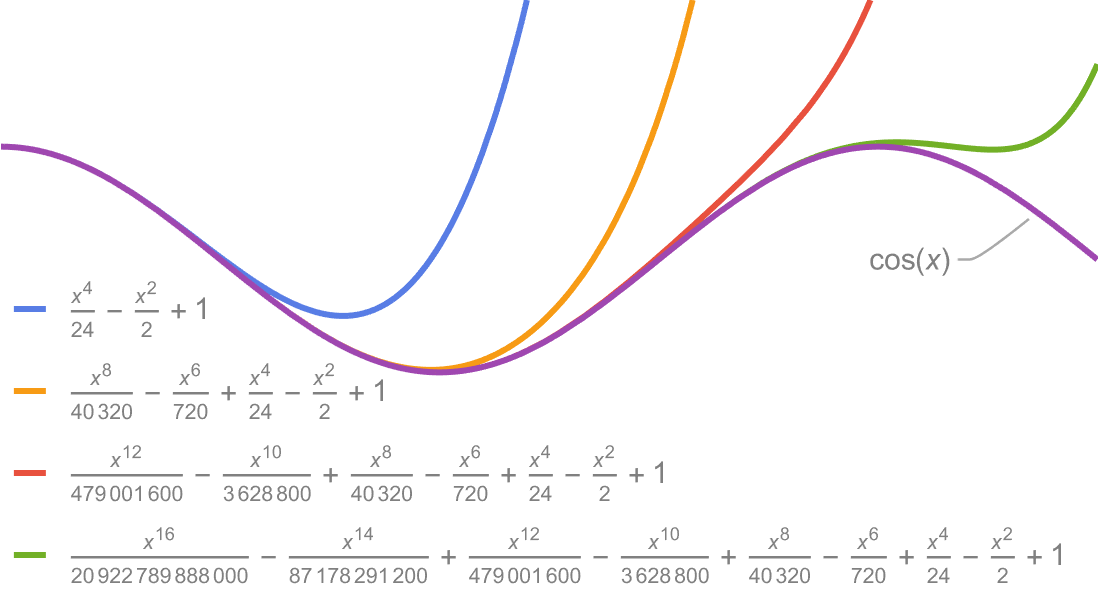## Integral & Summation Transforms

Transform data or functions to highlight different characteristics for applications in mathematics, physics and engineering. Symbolic and numeric Fourier, Laplace, Z and other advanced transforms such as Hankel, Radon and Mellin can help solve differential equations, analyze images and detect features in signals.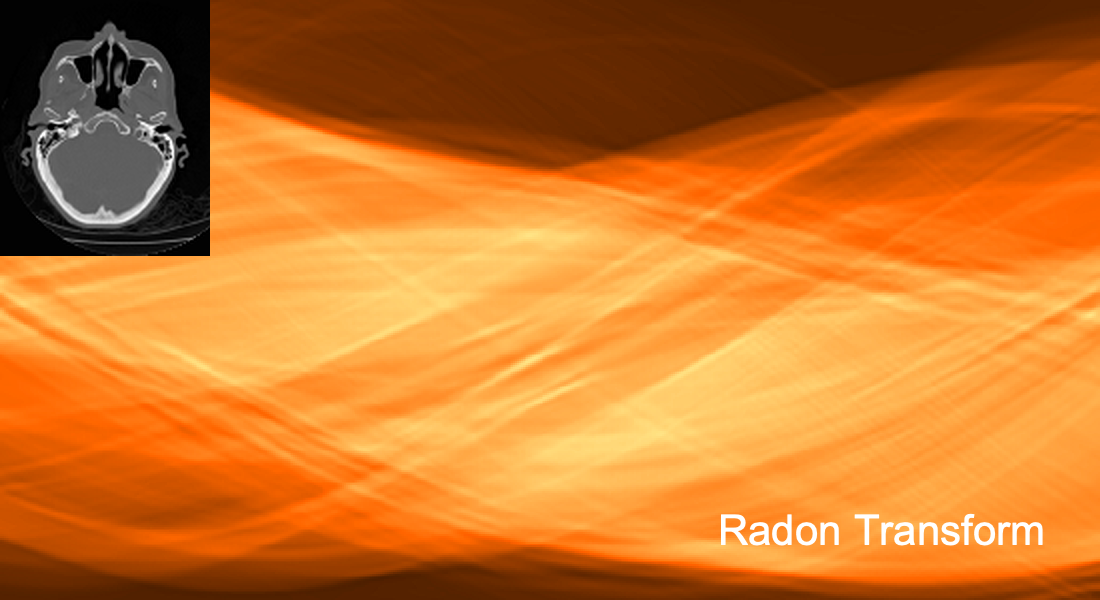## Wolfram Calculus & Algebra Documentation

Thousands of working examples, tutorials, guides and workflows

## Get Started with Wolfram Calculus & Algebra### Access the Wolfram Language throughWolfram|One or Mathematica

The state of the art in technical computing and the principal programming software for millions of innovators, educators and students around the world.### Get Help with Your Project

Enlist the world's computation experts to help with your project, no matter its size.